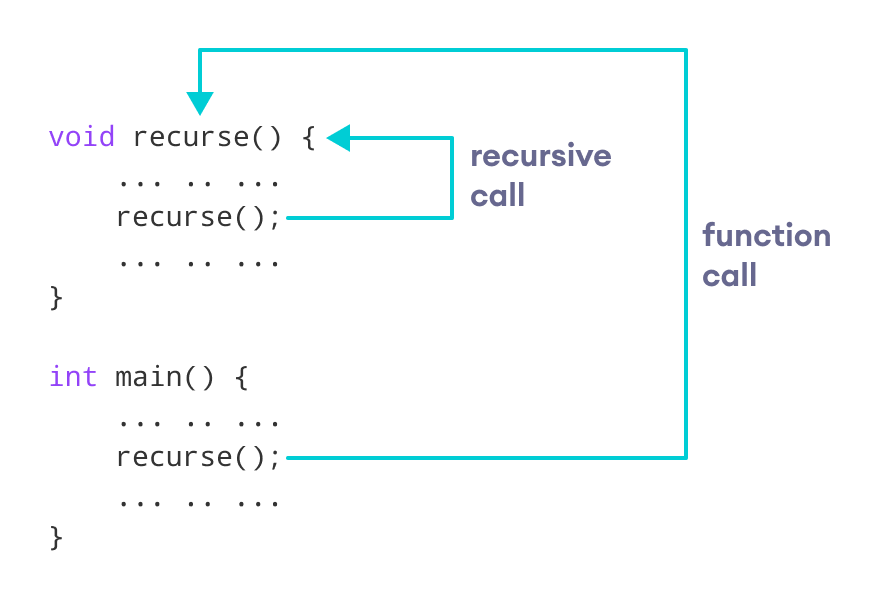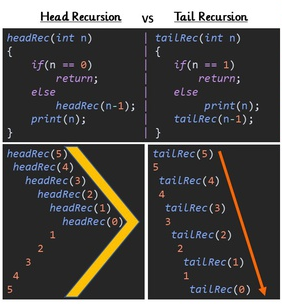# Learning RECURSION isn't so Recursive

### Hello World 👋

In this article, we will walk over through a complete guide to learn Recursion. Recursion is one of the major concepts for competitive coding as well as for placement. After completing this article and the resources shared in this article you will have a good understanding of Recursion.

### Goal :

1. What is Recursion?
2. What is a Call Stack in Recursion?
3. Convert ANY Iterative method to a Recursive method

Example 1 : Factorial Using Recursion Example 2 : Reverse an Array Using Recursion Example 3 : Bubble Sort Using Recursion Reducible Tutorial : Steps to solve ANY recursive problem Insidecode Tutorial : Use recursion to solve ANY binary tree problem ApniKaksha Tutorial : Recursion Simplified in 3 Steps

4. Time & Space Complexity of a Recursive Algorithm

5. Application of Recursion

a) Binary Search b) Fibonacci Sequence c) Tower of Hanoi d) Reversing a Linked List via recursion e) Binary Tree Traversal (Pre-Order/In-Order/Post-Order) f) Merge Sort g) Quick Sort h) Depth First Search for a Graph

6. Practice Problems

7. Is recursion really worth using?

a) Iteration vs Recursion b) Head vs Tail Recursion c) Why Stack Overflow error occurs in Recursion?

8. What after Recursion?

### 1) What is Recursion?

This is how Google explains recursion :A wise man once said :

“In order to understand recursion, one must first understand recursion.”

Confused? Well... Recursion can be tough to understand, especially for beginners. In its simplest form, a recursive function is the one that calls itself.Think of it this way, if you want to repeat the same set of operations present in the function, then instead of using a "for" loop or a "while" loop you have the option to call the function itself.Deep dive in recursion by following resources below: a) Understanding Recursion (by azamsharp)

Recursive functions can be classified as follows :

• Direct / Indirect : If the functions call itself directly or indirectly
• Head / Tail : If an operation is pending at each recursive call
• Linear / Tree : Based on the structure of the function calling pattern

### 2) What is a Call Stack in Recursion?

Recursive functions use something called the “call stack”.

After calling the function recursively, there can be several operations that are yet to be executed. Which makes it important for the system to set aside some space in memory for the function to resume once the recursive calls are finished executing. The space assigned for the recursive calls to resume their execution is known as the "Call Stack".

Here are some awesome explanations of "Call Stack" :

### 3) Convert ANY Iterative method to a Recursive method

We know that any iterative method can be converted to a recursive method. Because both of them basically perform a specific set of operation repeatedly (Click here to see that both iterative & recursive yields same output)

A general approach to convert ANY iterative function to a recursive function is by observing few properties of the iterative function.

• Step 1 : Identify the Base Case (Case that ends the iteration)
• Step 2 : Identify the Repeating Logic (Logic that gets executed in every iteration)
• Step 3 : Identify the Variables & its State (Variables that gets accessed on every iteration & its modification after every iteration ends)

Since each iteration is corresponding to each call stack in recursion, hence we need to make sure that the same logic and same set of variables are present in a recursive function.

Example 1 : Factorial Using Recursion

• Iterative Method :• Recursive Method :• Reference : a) GFG Editorial (Recursive + Iterative)

Example 2 : Reverse an Array Using Recursion

• Iterative Method :• Recursive Method :Example 3 : Bubble Sort Using Recursion

• Iterative Method :• Recursive Method :• Reference : a) GFG Editorial b) EDUFECT.COM Editorial c) Learners Bucket Editorial

Still, confused? Have a look at these tutorials which generalize the approach to come up with a recursive solution :

### 4) Time & Space Complexity of a Recursive Algorithm

a) CodesDope Editorial :

b) InterviewBit's Video Explanation :

c) Gate Lectures by Ravindrababu Ravula's Video Explanation :

d) Abdul Bari's Solving Recurrence Equations :

### 5) Application of Recursion

a) Binary Search (Link)

b) Fibonacci Sequence (Link)

c) Tower of Hanoi (Link)

d) Reversing a Linked List via recursion (Link)

e) Binary Tree Traversal (Pre-Order/In-Order/Post-Order)

f) Merge Sort (Link)

g) Quick Sort (Link)

h) Depth First Search for a Graph (Link)

### 6) Practice Problems

Here are some more collection of problems that you can refer to practice & have a good command over recursion:

### 7) Is recursion really worth using?

a) Iteration vs Recursion

• Following picture summarizes the difference :b) Head vs Tail Recursion

• Resource : JAVAAID Visual Explanationc) Why Stack Overflow error occurs in Recursion?

Recursion has greater space requirements as compared to Iteration because all the function calls must be stored in the recursion call stack to allow recursion to return back to the caller function.

The number of frames the recursion call stack can hold is finite. Now, when the stack can take no more frames, it is said to be overflowed. That is you get the infamous stack overflow exception.

It is important to learn to break things in order to prevent it from breaking. Let's get the stack overflow exception deliberately. XD

``````int factorial(int n)
{
if(n==1) return 1;

return n*factorial(n-1);
}

int main()
{
int a = 7;
cout << factorial(a);
}
``````

Call the above function with a very large number. Say a hundred thousand. So set `a = 100000` And that is how you overflow the stack.

### Wrap Up

Now you must be familiar with all the concepts of recursion. Practice problems on different platforms in order to master the art of recursion.

#Happy_Coding 💻

Article contributed by Kunal Chand

Thank you for reading the article. Follow CodoPedia for More. 😃

For regular updates and more interesting stuff Join us on Telegram